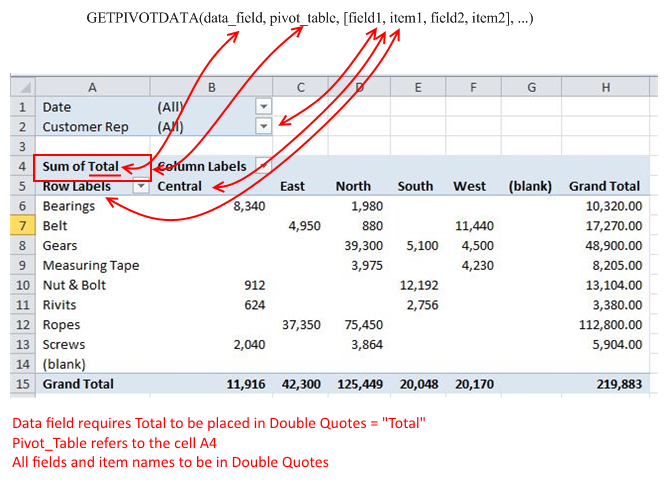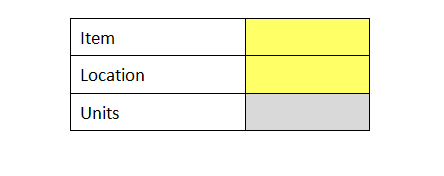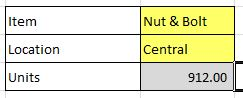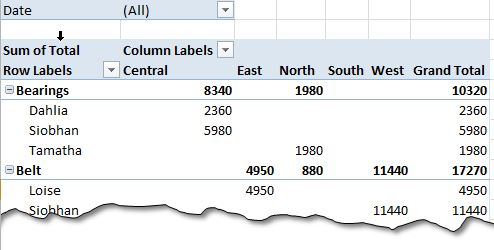# Getting Data From A Pivot Table – Using Getpivotdata()If you have a large pivot and want to create a report based on that pivot, it is time to revert to a function dedicated for it – GETPIVOTDATA().

GETPIVOTDATA() is function that can bring back the data from the pivot table, that was created using a list. It works like a lookup function, can have many more criteria’s for looking up for a value than a conventional VLOOKUP().

As we have been doing, we will use an example to see how we can use this formula and how we can create custom reports by using this formula:

### GETPIVOTDATA Syntax:

The following illustration gives the idea of the formula’s syntax and the corresponding element in the pivot table. It should be noted that data_field is the field that is being summed or count and the name of field should be in double quotes, similarly all the fields and items needed to be in double quotes. The pivot_table refers to the left and top most cell of the pivot. For this case it is A4.### Example:

Let’s assume we want to populate the following table based on a pivot table data.We will use data validation to populate the lists in the table and will try to fetch “Units”, based on the selection. We need to setup a GETPIVOTTABLE() function like this:

=GETPIVOTDATA("Total",Sheet4!A4,"Item",C2,"Location",C3)

The Syntax has already been explained in the preceding lines. We are looking for the sum of Total (Total Sales) for a Criteria in C2  for Item name and in C3 for the location.By selecting the criteria, the result is displayed automatically in the Units cell. The formula slices the table for the given criteria and returns the corresponding result. We can improve the number of criteria to get more insight. Look at the following table:In the above table, we have added another criterion to the column on right side – we have added the name of the sales representative to further breakup the data and we can keep rearranging data to get the desired results.